# sklearn.datasets.load_digits¶

sklearn.datasets.load_digits(n_class=10, return_X_y=False)[source]

Load and return the digits dataset (classification).

Each datapoint is a 8x8 image of a digit.

 Classes 10 Samples per class ~180 Samples total 1797 Dimensionality 64 Features integers 0-16

Read more in the User Guide.

Parameters: n_class : integer, between 0 and 10, optional (default=10) The number of classes to return. return_X_y : boolean, default=False. If True, returns (data, target) instead of a Bunch object. See below for more information about the data and target object. New in version 0.18. data : Bunch Dictionary-like object, the interesting attributes are: ‘data’, the data to learn, ‘images’, the images corresponding to each sample, ‘target’, the classification labels for each sample, ‘target_names’, the meaning of the labels, and ‘DESCR’, the full description of the dataset. (data, target) : tuple if return_X_y is True New in version 0.18. This is a copy of the test set of the UCI ML hand-written digits datasets http://archive.ics.uci.edu/ml/datasets/Optical+Recognition+of+Handwritten+Digits

Examples

To load the data and visualize the images:

>>> from sklearn.datasets import load_digits
>>> digits = load_digits()
>>> print(digits.data.shape)
(1797, 64)
>>> import matplotlib.pyplot as plt
>>> plt.gray()
>>> plt.matshow(digits.images)
>>> plt.show()


## Examples using sklearn.datasets.load_digits¶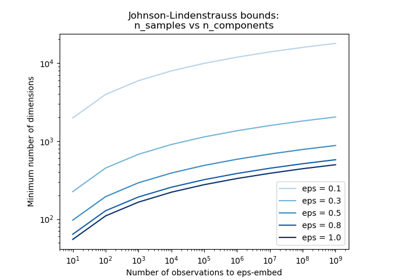The Johnson-Lindenstrauss bound for embedding with random projections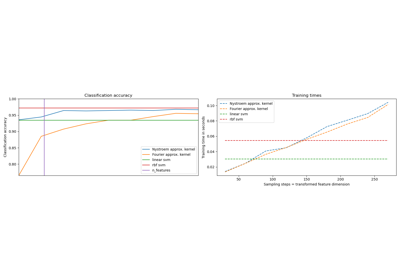Explicit feature map approximation for RBF kernels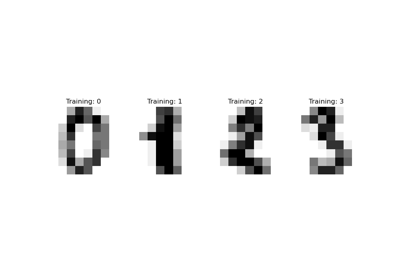Recognizing hand-written digits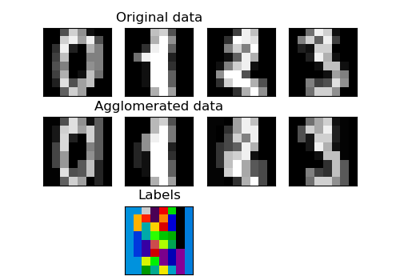Feature agglomeration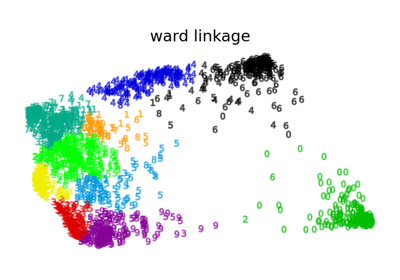Various Agglomerative Clustering on a 2D embedding of digits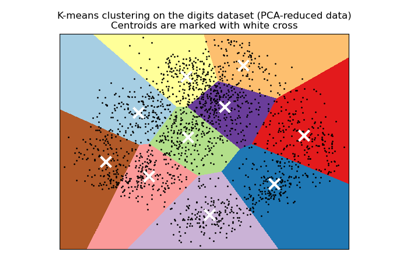A demo of K-Means clustering on the handwritten digits data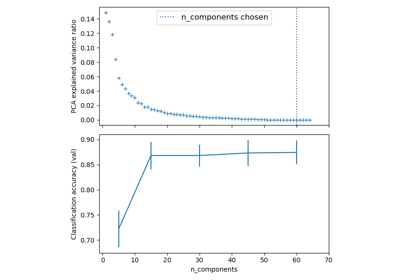Pipelining: chaining a PCA and a logistic regression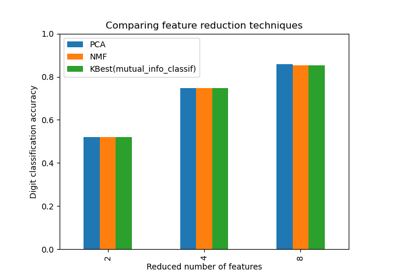Selecting dimensionality reduction with Pipeline and GridSearchCVThe Digit Dataset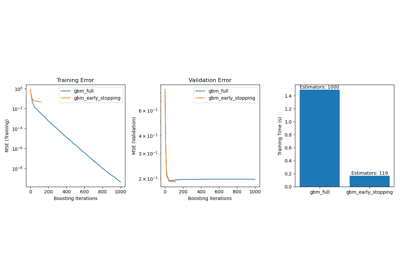Early stopping of Gradient BoostingDigits Classification ExerciseCross-validation on Digits Dataset Exercise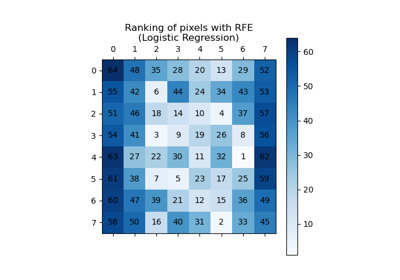Recursive feature eliminationComparing various online solvers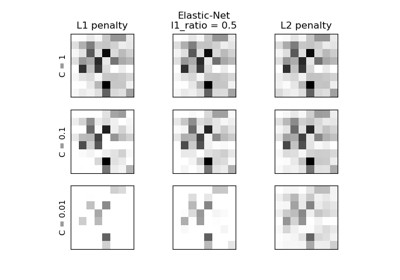L1 Penalty and Sparsity in Logistic Regression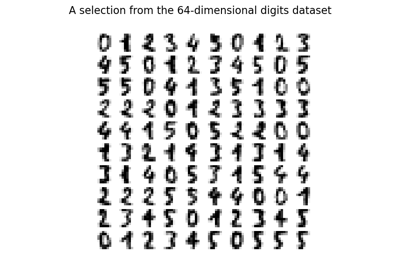Manifold learning on handwritten digits: Locally Linear Embedding, Isomap…Plotting Validation CurvesParameter estimation using grid search with cross-validationComparing randomized search and grid search for hyperparameter estimation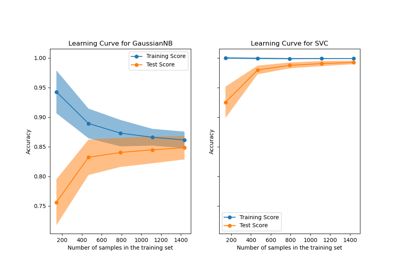Plotting Learning Curves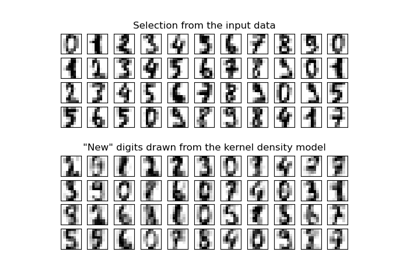Kernel Density Estimation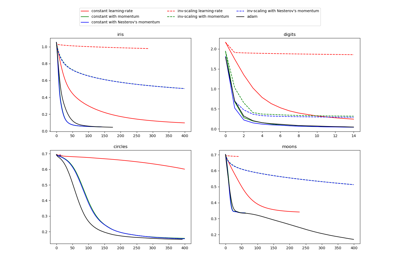Compare Stochastic learning strategies for MLPClassifier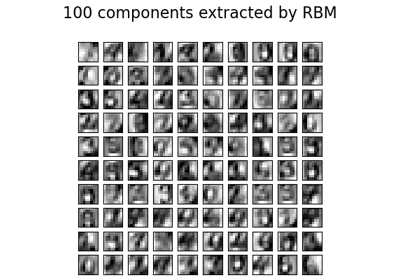Restricted Boltzmann Machine features for digit classification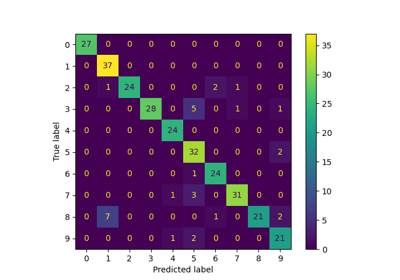Label Propagation digits: Demonstrating performance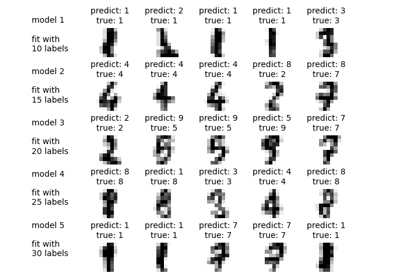Label Propagation digits active learning